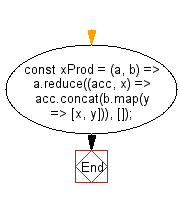# JavaScript: Create a new array out of the two supplied by creating each possible pair from the arrays

## JavaScript fundamental (ES6 Syntax): Exercise-25 with Solution

Write a JavaScript program to create a new array out of the two supplied by creating each possible pair from the arrays.

• Use Array.prototype.reduce(), Array.prototype.map() and Array.prototype.concat() to produce every possible pair from the elements of the two arrays.

Sample Solution:

JavaScript Code:

``````//#Source https://bit.ly/2neWfJ2
const xProd = (a, b) => a.reduce((acc, x) => acc.concat(b.map(y => [x, y])), []);
console.log(xProd([1, 2], ['a', 'b']));
console.log(xProd([1, 2], [1, 2]));
console.log(xProd(['a', 'b'], ['a', 'b']));
```
```

Sample Output:

```[[1,"a"],[1,"b"],[2,"a"],[2,"b"]]
[[1,1],[1,2],[2,1],[2,2]]
[["a","a"],["a","b"],["b","a"],["b","b"]]
```

Pictorial Presentation:Flowchart:Live Demo:

See the Pen javascript-basic-exercise-1-25 by w3resource (@w3resource) on CodePen.

Improve this sample solution and post your code through Disqus

What is the difficulty level of this exercise?

Test your Programming skills with w3resource's quiz.

﻿

## JavaScript: Tips of the Day

Chunks an array into n smaller arrays

Example:

```const tips_chunkIntoN = (arr, n) => {
const size = Math.ceil(arr.length / n);
return Array.from({ length: n }, (v, i) =>
arr.slice(i * size, i * size + size)
);
}
console.log(tips_chunkIntoN([1, 2, 3, 4, 5, 6, 7,8], 4));
```

Output:

```[[1,2],[3,4],[5,6],[7,8]]
```